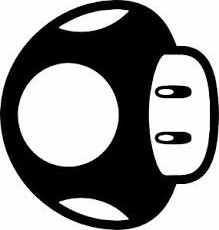# When carve begins, the Y axis goes off the work space and the job is ran sideways?

I built a CNC with TB6560 drivers, nema 23’s, GRBL v 1.1, and Easel. I calibrated the machine and all seemed well. Once the carving began the Y axis traveled off of my workspace in several places but then noticed that the carving I did was sideways so that explained the Y axis going offf my workspace problem but…why is my my machine carving the job sideways instead of how it displays in the visualizer?

@JasonMccollum

A schematic diagram if you designed it, manufacturer and model if you bought it, or lots of pictures if you just built it.

What software are you using to generate the file to carve and which program are you using to send the code to your machine, which OS on the computer?

@LarryM Sorry I should know better working as a programmer. Windows, Easel using Chrome as gcode sender. 3 Nema 23, rated at 3amps, Using Arduino Uno and TB6560 drivers. Flashed the Arduino with Grbl V 1.1 and Using the newest Easel diver. The baud rate for my port, the Arduino IDE and Easel is 115200. I jogged the motors and each axis worked properly. The X axis stepper is under the work area and Y, Z axis motors are shown in one of the pics. In the cooling box I built. the Drivers from the left of the photo go in order Z, Y, X I took some photos.

Thanks again.

Using the Arduino IDE serial monitor enter the command \$\$ and report the results here.

Will do. Give me a few minutes to run out to the shop.

So, the gantry going left/right is your Y axis and your X axis is moving the table/gantry forward and backwards?

If so, that’s reverse of the Xcarve (and probably most other CNC) which Easel was designed for and explains your design rotation.

Here’s the settings. I should mention I have configured this for 1/2 step currently.

ok
\$132=100.000
\$131=790.000
\$130=790.000
\$122=50.000
\$121=50.000
\$120=50.000
\$112=6400.000
\$111=6400.000
\$110=6400.000
\$102=5.000
\$101=5.000
\$100=5.000
\$32=0
\$31=0
\$30=12000
\$27=1.000
\$26=250
\$25=750.000
\$24=25.000
\$23=3
\$22=0
\$21=0
\$20=0
\$13=0
\$12=0.002
\$11=0.020
\$10=115
\$6=0
\$5=0
\$4=0
\$3=7
\$2=0
\$1=0
\$0=10
\$\$
ok
\$102=5
ok
\$132=100.000
\$131=790.000
\$130=790.000
\$122=50.000
\$121=50.000
\$120=50.000
\$112=6400.000
\$111=6400.000
\$110=6400.000
\$102=188.976
\$101=5.000
\$100=5.000
\$32=0
\$31=0
\$30=12000
\$27=1.000
\$26=250
\$25=750.000
\$24=25.000
\$23=3
\$22=0
\$21=0
\$20=0
\$13=0
\$12=0.002
\$11=0.020
\$10=115
\$6=0
\$5=0
\$4=0
\$3=7
\$2=0
\$1=0
\$0=10
\$\$
ok
\$132=100.000
\$131=790.000
\$130=790.000
\$122=50.000
\$121=50.000
\$120=50.000
\$112=6400.000
\$111=6400.000
\$110=6400.000
\$102=188.976
\$101=5.000
\$100=5.000
\$32=0
\$31=0
\$30=12000
\$27=1.000
\$26=250
\$25=750.000
\$24=25.000
\$23=3
\$22=0
\$21=0
\$20=0
\$13=0
\$12=0.002
Events
Settings
\$0=10
\$1=0
\$2=0
\$3=7
\$4=0
\$5=0
\$6=0
\$10=115
\$11=0.020
\$12=0.002
\$13=0
\$20=0
\$21=0
\$22=0
\$23=3
\$24=25.000
\$25=750.000
\$26=250
\$27=1.000
\$30=12000
\$31=0
\$32=0
\$100=5.000
\$101=5.000
\$102=5.000
\$110=6400.000
\$111=6400.000
\$112=6400.000
\$120=50.000
\$121=50.000
\$122=50.000
\$130=790.000
\$131=790.000
\$132=100.000

Yes left/right is Y axis and X axis is front/backwards. So Xcarve is setup typically to have the left right being X axis? I did not know that.

If you are using Easel to do your design work then you are going to have to make your machine look like an X-carve because Easel does some things behind the scenes that might be messing you up.

If you are using something other than Easel for your CAM work you don’t need Easel, but can use one of the other G-code sending programs.

@LarryM You’re correct. In the visualizier the project looks right side up like the first image but carves like the 2nd…Thought about using UGS but Easel just seemed a bit easier. I didn’t design it. Just import SVG and let Easel do the Gcode

@JasonMccollum
Ok. while you’re in there change these things for 1.1f/g

\$1=255
\$10=1
\$13=1 only if you want grbl to report in inches

When you get things running consider:

\$20=1 use soft limit — \$22 must be set before \$20

\$22= 1 use homing – only if you have homing switches

You may need to work up a set of custom speeds and acc.

Make sure that D11 is used for Variable Spindle speed PWM and D12 is Homing switch Z

Thanks so much. I don’t have homing switches but will try those settings.

The idea behind Easel was a good one. but implementation is some what lacking, I think they underestimated the resources required for the software side of things.

3 Likes